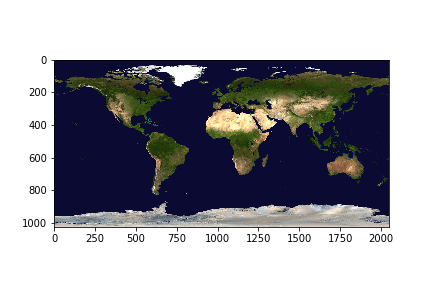GeeksforGeeks App
Open AppBrowser
Continue

# Visualizing Tiff File Using Matplotlib and GDAL using Python

Tiff file formats are used for storing raster images. A library called GDAL- Geospatial Data Abstraction Library is specially used for the purpose of reading such raster files along with other file formats like vector formats. The gdal module belongs to Open Source Geospatial Foundation

To install this module run this command into your terminal.

```pip install GDAL
```

To visualize a tiff file we need matplotlib and GDAL modules in python.

#### Approach:

1. Import the module
2. Count the number of bands.
3. Fetch all the raster bands from the tiff file.
4. Read the bands into NumPy arrays.
5. Pass the arrays into Matplotlib’s imshow() to visualize.

#### Step-by-step implementation:

Step 1: Import the modules and open the file.

## Python3

 `from` `osgeo ``import` `gdal``import` `matplotlib.pyplot as plt`` ` ` ` `dataset ``=` `gdal.``Open``(r``'land_shallow_topo_2048.tif'``)`

Step 2: Count the number of bands.

## Python3

 `print``(dataset.RasterCount)`

Output:

```3
```

Step 3: Fetch the bands,

To fetch the bands we use GDAL’s GetRasterBand(int).
Note that the value of int we pass will always start from 1 (indexing of bands starts from 1),

## Python3

 `# since there are 3 bands``# we store in 3 different variables``band1 ``=` `dataset.GetRasterBand(``1``) ``# Red channel``band2 ``=` `dataset.GetRasterBand(``2``) ``# Green channel``band3 ``=` `dataset.GetRasterBand(``3``) ``# Blue channel`

Step 4: Read the bands as Numpy arrays.

GDAL provides ReadAsArray() method that converts the bands into numpy arrays and returns them.

## Python3

 `b1 ``=` `band1.ReadAsArray()``b2 ``=` `band2.ReadAsArray()``b3 ``=` `band3.ReadAsArray()`

Step 5: Plotting the arrays using imshow().

To plot the three arrays we will stack them in sequence.

## Python3

 `img ``=` `np.dstack((b1, b2, b3))``f ``=` `plt.figure()``plt.imshow(img)``plt.savefig(``'Tiff.png'``)``plt.show()`

Output:My Personal Notes arrow_drop_up